# Superconducting qubits: improving gate robustness using digital control

Generating single flux quantum gates robust to leakage and frequency drift

BOULDER OPAL enables you to optimize and simulate quantum systems subjected to a wide range of pulsed controls. This application note considers controls based on Single Flux Quantum (SFQ) digital logic, consisting of trains of ultrafast flux pulses applied at rates comparable to the qubit frequency.

SFQ circuitry can be embedded in the proximity of the quantum processing unit, as illustrated in the figure below. This helps circumventing issues that can arise from the room-temperature electronics used to generate microwave control pulses. However, the digitized nature of such controls opens up new challenges, such as susceptibility to leakage to other levels and frequency drifts. In this application note, you’ll address these issues by designing the spacing between individual pulses, constructing sequences that are robust to leakage and frequency detuning.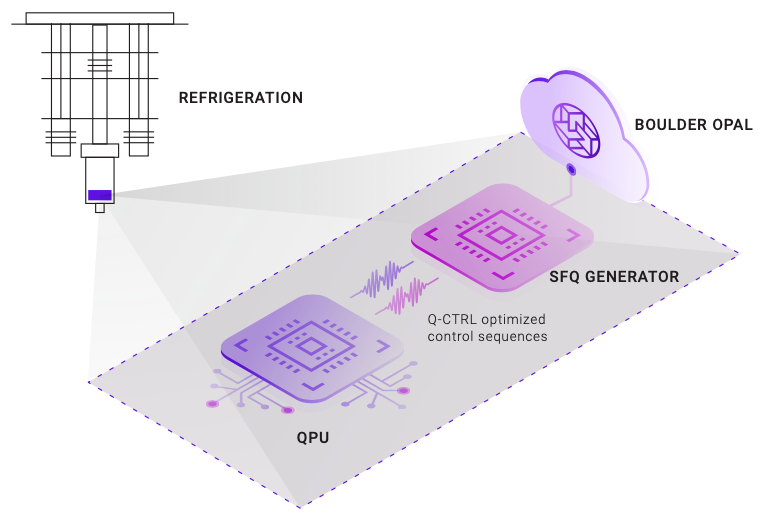The notebook is organized as follows: In the first section, you'll explore the standard SFQ gates, which feature a train uniformly spaced pulses at the rate equal to that of the qubit frequency. In the second section, you'll use BOULDER OPAL to generate robust SFQ gates by optimizing the arrival time of the pulses in the sequence.

## Imports and initialization

# BOULDER OPAL
from qctrl import Qctrl

qctrl = Qctrl()

import numpy as np
import matplotlib.pyplot as plt
from qctrlvisualizer import plot_controls
from qctrlvisualizer import get_qctrl_style
from scipy.linalg import expm

plt.style.use(get_qctrl_style())

# helper function
def sequence_times_to_control_format(pulse_times, gate_duration):
"""
Takes in the sequence of SFQ pulse times constituting a gate of total duration set by gate_duration
and outputs the control sequences describing qubit frequency omega, anharmonicity alpha and SFQ Rabi
rate Omega_eff for each pwc segment.
SFQ pulses are represented as square segments where pulse_times define the start of each pulse.
"""
segment_durations = pulse_times[1:] - pulse_times[0:-1]
segment_durations = np.append(segment_durations, [gate_duration - pulse_times[-1]])

control_durations = np.array([pulse_times])
# binary switch to mark the segments where the square SFQ pulses are turned on
control_switch = np.array()
for segment_duration in segment_durations:
# encoding for pulse controls (1 during pulse duration, 0 otherwise)
control_durations = np.append(
control_durations, [pulse_duration, segment_duration - pulse_duration]
)
control_switch = np.append(control_switch, [1.0, 0.0])

return {
"omegas": [
{
"duration": gate_duration,
"value": omega,
}
],
"alphas": [
{
"duration": gate_duration,
"value": alpha,
}
],
"pulses": [
{
"duration": d,
"value": v,
}
for d, v in zip(control_durations, control_switch * Omega_eff)
],
}

def evolve_states(initial_state, controls, sample_times):
"""
Takes in the initial qubit state, the controls as defined by sequence_times_to_control_format and
outputs the sequence of state evolution at sample_times.
"""

omega_values = np.array([s["value"] for s in controls["omegas"]])
omega_durations = np.array([s["duration"] for s in controls["omegas"]])

alpha_values = np.array([s["value"] for s in controls["alphas"]])
alpha_durations = np.array([s["duration"] for s in controls["alphas"]])

pulse_values = np.array([s["value"] for s in controls["pulses"]])
pulse_durations = np.array([s["duration"] for s in controls["pulses"]])

with qctrl.create_graph() as graph:

# hamiltonian terms
omega_signal = qctrl.operations.pwc(
values=omega_values, durations=omega_durations
)
alpha_signal = qctrl.operations.pwc(
values=alpha_values, durations=alpha_durations
)
pulse_signal = qctrl.operations.pwc(
values=pulse_values, durations=pulse_durations
)
hamiltonian = (
omega_signal * ada + alpha_signal / 2 * ad2a2 + pulse_signal * (a + ad) / 2
)

# calculate the evolution unitaries
unitaries = qctrl.operations.time_evolution_operators_pwc(
hamiltonian=hamiltonian, sample_times=sample_times
)

evolved_states = unitaries @ initial_state[:, None]
evolved_states.name = "evolved_states"

graph_result = qctrl.functions.calculate_graph(
graph=graph, output_node_names=["evolved_states"]
)

return graph_result.output["evolved_states"]["value"]

schemes = ["Standard", "Q-CTRL"]
colors = {
"Standard": "#000000",
"Q-CTRL": "#680CE9",
"state0": "#250D2E",
"state1": "#7B7479",
"state2": "#BF04DC",
}

# data holders for SQF pulse sequences
gate_durations = {}
pulse_times = {}
controls = {}


## Standard SFQ gate

In this section, you'll set up the standard $X$ gate based on a train of uniformly spaced SFQ pulses set apart by one Larmor period of the qubit ground state, described by the following Hamiltonian:

$$H = (\omega + \Delta) a^\dagger a + \frac{\alpha}{2} (a^\dagger)^2 a^2 + \frac{\Omega (t)}{2}\left(a + a^\dagger\right),$$

where $a$ is the lowering operator, $\omega$ is the transmon frequency, $\Delta$ the frequency drift, $\alpha$ the anharmonicity, and $\Omega (t)$ the train of SFQ pulses.

You'll now construct an $X$ gate of duration $d_{\text{gate}} = 10\,$ns composed of $n=50$ SFQ pulses at times $t_k =k \frac{2\pi}{\omega}$, $k\in [0,1,... n-1]$, where each pulse perform a rotation of $\theta = \pi/50$.

As the SFQ pulse duration $d_{\text{pulse}}=10\,$ps is short relative to the precession of the qubit, $d_{\text{pulse}}\ll\frac{2\pi}{\omega}$, you can conveniently model $\Omega (t)$ as a sequence of square pulses of equal amplitude $\Omega_{\text{eff}}=\theta/d_{\text{pulse}}$, as follows: $$\Omega (t) = \begin{cases} \Omega_{eff} & \quad t \in (t_k, t_k + d_{\text{pulse}}) \\ 0 & \quad \text{otherwise} \end{cases}.$$

In the code below, you'll set up the "standard" $X$ gate and evaluate its state evolution using a three-level representation of the transmon.

# system parameters
alpha = -2 * np.pi * 400e6  # rad.Hz, transmon anharmonicity
omega = 2 * np.pi * 5e9  # rad.Hz, transmon frequency

a = np.array(
[[0.0, 1.0, 0.0], [0.0, 0.0, np.sqrt(2)], [0.0, 0, 0.0]], dtype="complex128"
)
ad = a.conj().T
ada = ad @ a
ad2a2 = ad @ ad @ a @ a

# SFQ pulse parameters
pulse_duration = 10e-12  # s
theta = np.pi / 50
Omega_eff = theta / pulse_duration

# define the standard sequence of pulses (pulse rate is equal to transmon frequency)
pulse_count = 50
gate_durations["Standard"] = 10e-9  # s
pulse_times["Standard"] = np.arange(0, pulse_count) * 2 * np.pi / omega

controls["Standard"] = sequence_times_to_control_format(
pulse_times["Standard"], gate_durations["Standard"]
)

# plot the controls
plot_scheme = "Standard"
print(
plot_scheme, "gate duration:", np.round(gate_durations[plot_scheme] * 1e9, 2), "ns"
)
fig = plt.figure()
fig.suptitle(plot_scheme + " SFQ pulse sequence", fontsize=16, y=1.0)
plot_controls(
figure=fig,
controls={"$\Omega(t)$": controls[plot_scheme]["pulses"]},
)
fig.set_size_inches(16, 2)
plt.gca().get_lines().set_color(colors["Standard"])

Standard gate duration: 10.0 ns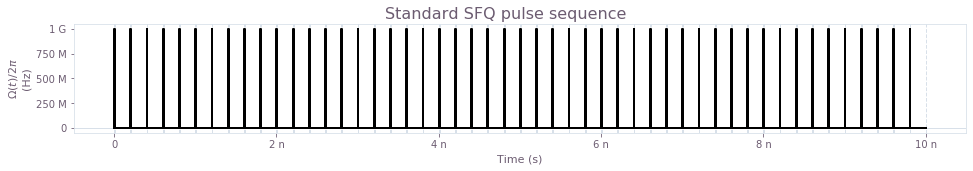Plotted is the sequence of square SFQ pulses representing the standard $X$ gate. Here the spacing between the single flux pulses is set equal to the transmon frequency.

initial_state = np.array([1, 0, 0])
sample_times = np.linspace(0, gate_durations["Standard"], 100)

state_evolution = evolve_states(initial_state, controls["Standard"], sample_times)

Your task calculate_graph has completed in 3s.


fig, ax1 = plt.subplots()
plt.xlabel("Time (ns)", fontsize=14)
plt.title("State evolution", fontsize=16)

p0 = ax1.plot(
sample_times * 1e9,
np.abs(state_evolution[:, 0]) ** 2,
label="$P_{0}$",
color=colors["state0"],
)
p1 = ax1.plot(
sample_times * 1e9,
np.abs(state_evolution[:, 1]) ** 2,
label="$P_{1}$",
color=colors["state1"],
)
ax1.set_ylabel("Probability $P_{0},P_{1}$", fontsize=14)
ax1.tick_params(labelsize=14)

ax2 = ax1.twinx()
p2 = ax2.plot(
sample_times * 1e9,
np.abs(state_evolution[:, 2]) ** 2,
label="$P_{2}$",
color=colors["state2"],
)

ax2.set_yscale("log")
ax2.set_ylim([1e-8, 10])
ax2.set_ylabel("Probability $P_{2}$", fontsize=14)
ax2.tick_params(labelsize=14)

lgns = p0 + p1 + p2
lbs = [l.get_label() for l in lgns]
plt.legend(lgns, lbs, loc="center left", fontsize=14)
plt.show()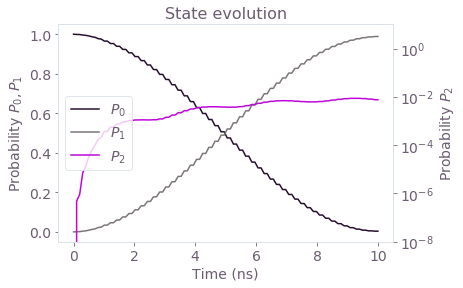Evolution of the populations of the three transmon levels during the application of the standard SFQ $X$ gate for the system initialized in the ground state. The populations for the qubit states $0$ and $1$ are shown in a linear scale (left axis) while the smaller population of state $2$ is shown in the log scale (right axis). Note that the leakage to the third state induces a significant operational infidelity ($I \sim 10^{-2}$).

## Optimized SFQ gate

In this section, you'll use BOULDER OPAL to generate an SFQ gate robust to both leakage and the drift in the qubit frequency. You will do this by allowing the application time of each pulse to vary. In experiments, the pulse time $t_k$ commonly changes discretely, in increments defined by a clock rate $\omega_c$. However, in the present example you'll make a simplifying assumption that the clock rate is significantly greater than the qubit frequency $\omega_c\gg\omega$. This allows the free precession periods between the pulses $\delta_t = t_{k+1}-t_k$ to vary continuously $\delta_t\in (0,1)\frac{2\pi}{\omega}$.

Leakage suppression is achieved by including the third energy level into the Hamiltonian and by providing the ideal $X$ gate qubit unitary as the target operation to the optimizer. Robustness to drift is achieved by including a Hamiltonian noise term corresponding to the energy variation between the first two transmon levels.

In the cells below, you will optimize an SFQ gate, plot its evolution and finally compare its performance against the standard gate from the previous section.

# ideal X gate target unitary
target = np.array([[0, 1, 0], [1, 0, 0], [0, 0, 0]])
projector = np.diag([1, 1, 0])

optimization_pulse_count = 160
optimization_count = 20

with qctrl.create_graph() as graph:

# binary switch to mark the segments where Omega_eff is turned on
control_switch = np.tile([1.0, 0.0], optimization_pulse_count)

# for convenience, encode signal pwc values in units of phase by setting the
# effetive duration of all pwc segments to 1; use number of segments as the effective gate duration
segment_count = len(control_switch)  #  SFQ + free precession segments

# omega component during the SFQ pulse
pulse_omega_signal = qctrl.operations.pwc_signal(
values=omega * pulse_duration * control_switch, duration=segment_count
)
# alpha component during the SFQ pulse
pulse_alpha_signal = qctrl.operations.pwc_signal(
values=alpha * pulse_duration * control_switch, duration=segment_count
)
# SFQ pulse
Omega_eff_signal = qctrl.operations.pwc_signal(
values=Omega_eff * pulse_duration * control_switch, duration=segment_count
)
# total pulse controls
pulse_terms = (
pulse_omega_signal * ada
+ pulse_alpha_signal / 2 * ad2a2
+ Omega_eff_signal * (ad + a) / 2
)

# define free precession durations to be optimization variable
free_durations = qctrl.operations.optimization_variable(
count=optimization_pulse_count,
lower_bound=0.0,  #  min free precession duration
upper_bound=2 * np.pi / omega,  # max free precession duration
name="free_durations",
)
# repeat elements to match the total number of segments, then switch off during the SFQ pulse segments
free_durations = qctrl.operations.repeat(free_durations, repeats=2, axis=0) * (
1.0 - control_switch
)
# omega free precession terms
free_omega_signal = qctrl.operations.pwc_signal(
values=omega * free_durations, duration=segment_count
)
# alpha free precession terms
free_alpha_signal = qctrl.operations.pwc_signal(
values=alpha * free_durations, duration=segment_count
)
free_precession_terms = free_omega_signal * ada + free_alpha_signal / 2 * ad2a2

# total hamiltonian term
hamiltonian = pulse_terms + free_precession_terms

# pysical duration of the gate: pulse durations + free presession durations
gate_duration = (
qctrl.operations.sum(free_durations) + optimization_pulse_count * pulse_duration
)
gate_duration.name = "gate_duration"

detuning_noise_signal = qctrl.operations.pwc_signal(
values=free_omega_signal.values
* 1.0
/ qctrl.operations.sum(
qctrl.operations.abs(free_omega_signal.values)
),  # normalized free precession phase values
duration=segment_count,
)
detuning_noise_term = detuning_noise_signal * np.array(
[[-1.0, 0.0, 0.0], [0.0, 1.0, 0], [0.0, 0, 0.0]], dtype="complex128"
)

# cost
cost = qctrl.operations.infidelity_pwc(
hamiltonian=hamiltonian,
target_operator=qctrl.operations.target(target @ projector),
noise_operators=[detuning_noise_term],
name="cost",
)
# gate infidelity
gate_infidelity = qctrl.operations.infidelity_pwc(
hamiltonian=hamiltonian,
target_operator=qctrl.operations.target(target),
name="gate_infidelity",
)

optimization_result = qctrl.functions.calculate_optimization(
graph=graph,
cost_node_name="cost",
output_node_names=["free_durations", "gate_duration", "gate_infidelity"],
optimization_count=optimization_count,
)

print("Optimization cost:", optimization_result.cost)
print(
"Operational infidelity :", optimization_result.output["gate_infidelity"]["value"]
)

optimized_free_durations = optimization_result.output["free_durations"]["value"]

pulse_times["Q-CTRL"] = np.arange(
0, optimization_pulse_count
) * pulse_duration + np.concatenate([, np.cumsum(optimized_free_durations[:-1])])

gate_durations["Q-CTRL"] = optimization_result.output["gate_duration"]["value"]

controls["Q-CTRL"] = sequence_times_to_control_format(
pulse_times["Q-CTRL"], gate_durations["Q-CTRL"]
)

Your task calculate_optimization has started.
Your task calculate_optimization has completed in 39s.
Optimization cost: 7.653080368409478e-05
Operational infidelity : 4.9398092394348225e-08

# plot the controls
plot_scheme = "Q-CTRL"
print(
plot_scheme, "gate duration:", np.round(gate_durations[plot_scheme] * 1e9, 2), "ns"
)
fig = plt.figure()
fig.suptitle(plot_scheme + " SFQ pulse sequence", fontsize=16, y=1.0)
plot_controls(
figure=fig,
controls={"$\Omega(t)$": controls[plot_scheme]["pulses"]},
)
fig.set_size_inches(16, 2)

Q-CTRL gate duration: 20.03 ns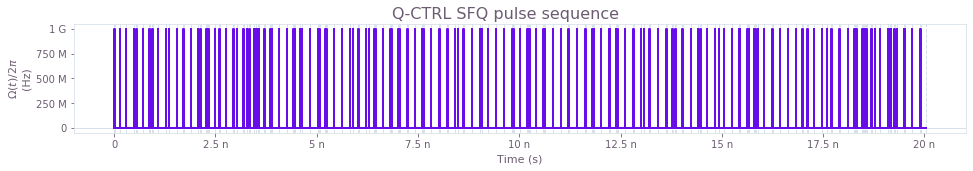Pulse sequence for the optimized SFQ $X$ gate. Note that using variable pulse spacing allows the optimizer to exploit more degrees of freedom in order to mitigate the effects of leakage and drift, as indicated by a more complex state evolution below.

initial_state = np.array([1, 0, 0])
sample_times = np.linspace(0, gate_durations["Q-CTRL"], 100)

optimized_state_evolution = evolve_states(
initial_state, controls["Q-CTRL"], sample_times
)

Your task calculate_graph has completed in 3s.


fig, ax1 = plt.subplots()
plt.xlabel("Time (ns)", fontsize=14)
plt.title("State evolution", fontsize=16)

p0 = ax1.plot(
sample_times * 1e9,
np.abs(optimized_state_evolution[:, 0]) ** 2,
label="$P_{0}$",
color=colors["state0"],
)
p1 = ax1.plot(
sample_times * 1e9,
np.abs(optimized_state_evolution[:, 1]) ** 2,
label="$P_{1}$",
color=colors["state1"],
)
ax1.set_ylabel("Probability $P_{0},P_{1}$", fontsize=14)
ax1.tick_params(labelsize=14)

ax2 = ax1.twinx()
p2 = ax2.plot(
sample_times * 1e9,
np.abs(optimized_state_evolution[:, 2]) ** 2,
label="$P_{2}$",
color=colors["state2"],
)

ax2.set_yscale("log")
ax2.set_ylim([1e-9, 100])
ax2.set_ylabel("Probability $P_{2}$", fontsize=14)
ax2.tick_params(labelsize=14)

lgns = p0 + p1 + p2
lbs = [l.get_label() for l in lgns]
plt.legend(lgns, lbs, loc="center left", fontsize=14)
plt.show()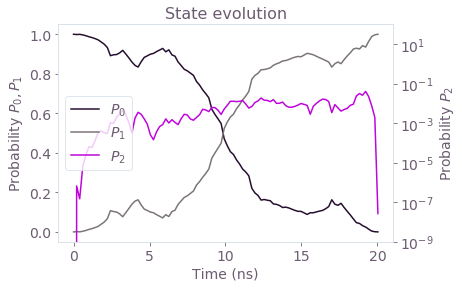Here is the evolution of the optimized SFQ X gate for the qubit initialized in the ground state. As in the plot for the standard gate, the population of the third level is shown in a log scale (right axis). Note that by the end of the gate, this population is minimized, allowing the operational infidelity ($I<10^{-7}$) to improve by 6 orders of magnitude as compared to the standard gate.

To demonstrate the gate robustness, in the cell below you'll perform a quasi-static scan by computing the gate infidelity over a range of detunings from the qubit frequency.

detunings = 2 * np.pi * np.linspace(-20, 20, 200) * 1e6
drift_infidelities = {}
for scheme in ["Standard", "Q-CTRL"]:
scan_controls = controls[scheme]

omega_values = np.array([s["value"] for s in scan_controls["omegas"]]) * np.ones(
[len(detunings), 1]
)
omega_durations = np.array([s["duration"] for s in scan_controls["omegas"]])

alpha_values = np.array([s["value"] for s in scan_controls["alphas"]]) * np.ones(
[len(detunings), 1]
)
alpha_durations = np.array([s["duration"] for s in scan_controls["alphas"]])

pulse_values = np.array([s["value"] for s in scan_controls["pulses"]]) * np.ones(
[len(detunings), 1]
)
pulse_durations = np.array([s["duration"] for s in scan_controls["pulses"]])

# set up the graph for the quasi-static scan simulation
with qctrl.create_graph() as graph:

omega_signal = qctrl.operations.pwc(
values=omega_values,
durations=omega_durations,
time_dimension=1,
)
detuning_signal = qctrl.operations.pwc_signal(
values=detunings[:, None],
duration=gate_durations[scheme],
)
alpha_signal = qctrl.operations.pwc(
values=alpha_values,
durations=alpha_durations,
time_dimension=1,
)
pulse_signal = qctrl.operations.pwc(
values=pulse_values,
durations=pulse_durations,
time_dimension=1,
)
hamiltonian = (
omega_signal * ada
+ alpha_signal / 2 * ad2a2
+ pulse_signal * (a + ad) / 2
+ detuning_signal * ada
)

# infidelities for detuning rates
infidelities = qctrl.operations.infidelity_pwc(
hamiltonian=hamiltonian,
target_operator=qctrl.operations.target(target),
name="infidelities",
)

# calculate the graph and extract infidelities
graph_result = qctrl.functions.calculate_graph(
graph=graph, output_node_names=["infidelities"]
)

drift_infidelities[scheme] = graph_result.output["infidelities"]["value"]

Your task calculate_graph has completed in 3s.


Your task calculate_graph has completed in 3s.


for scheme in schemes:
plt.plot(
detunings / (2 * np.pi * 1e6),
drift_infidelities[scheme],
color=colors[scheme],
label=scheme,
)
plt.xlabel("Frequency drift (MHz)", fontsize=14)
plt.ylabel("Infidelity", fontsize=14)
plt.title("Quasi-static scan", fontsize=16)
plt.tick_params(labelsize=14)
plt.legend(fontsize=14)
plt.ylim([0, 0.25])
plt.show()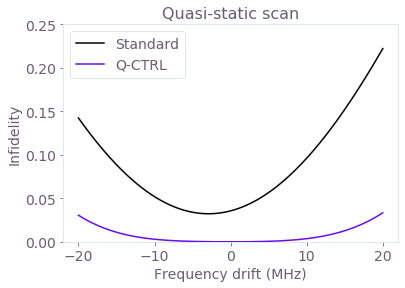Operational infidelity as a function of the drift in the qubit's frequency, allowing a comparison between the performances of the standard and the Q-CTRL gates. You can see the detrimental impact of leakage on the standard gate by its higher infidelity levels. In contrast, by minimizing leakage, the robust gate produces a much lower infidelity. Furthermore, due to the gate's robustness to frequency drift, this low infidelity level extends over a large range of detunings.